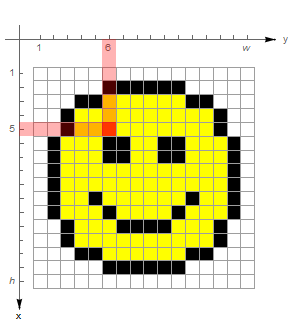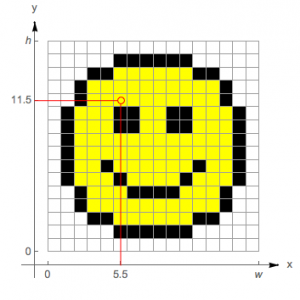# What image coordinate systems does the Wolfram Language use?

There are two common coordinate systems used in the field of image processing and analysis: the matrix coordinate system and the graphics coordinate system. They differ in how the locations of pixels are expressed.

### Matrix or index coordinates

The matrix coordinate space reflects the data matrix stored in the image. The matrix is stored in rows of columns of pixel values. Therefore, pixel locations are the same as their corresponding row and column in the data matrix, where rows run from top to bottom and columns run from left to right.

Pixels are therefore identified by integer coordinates, row number first. Coordinates {5,6} identify the red pixel in the image below. The x direction is down, and the y direction is across.In the Wolfram Language, functions that operate on both images and data arrays adhere to the matrix coordinate system. Examples include GaussianFilter, ImageConvolve and ImageData.

### Image or graphics coordinates

The origin of the image or graphics coordinate system is the bottom-left corner of an image. The x coordinate extends from left to right; the y coordinate extends upward.

Integers give the edges of pixels, like grid lines on graph paper.

An image object with dimensions of {width, height} is placed in the 2D space so that in a position {x,y} given in the standard image coordinate system, x runs continuously from 0 to width and y from 0 to height. The position {0,0} corresponds to the bottom-left corner. In the smiley image, the pixel that was at exactly {5,6} in matrix coordinates is now centered at {5.5,11.5} in image coordinates.Image pixels are covered by intervals between successive integer coordinate values. Thus, noninteger coordinates are inside a single pixel. Integer coordinates are located on pixel boundaries, however, and take all immediate pixel neighbors into account, either by selecting all neighboring pixels or by taking their average color value.

Wolfram Language functions that focus on images adhere to image coordinates. Examples include ImageValue, ImageCrop and ImageDimensions.

설명이 도움이 되었나요?

하실 말씀이 있습니까?

피드백 감사합니다.

## 지원 문의

청구서, 제품 동기화에 관한 질문에서 기술적인 질문까지 부담없이 문의하세요.전화하기

월요일 - 금요일
8am–5pm 중부 표준시

• 제품 등록 및 동기화
• 구매 전 정보 및 주문
• 설치 및 동작

## 고급 기술지원 (해당 고객을 대상으로)

월요일 - 목요일
8am–7pm 중부 표준시

금요일
8:30–10am & 11am–5pm 중부 표준시

• 우선적 기술지원
• Wolfram 전문가들의 제품 지원
• Wolfram 프로그래밍
• 고급 설치 지원